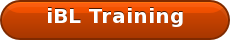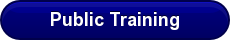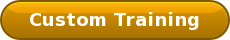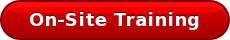Overall Equipment Effectiveness (OEE) is undoubtedly one of the best indicators of process reliability, but many struggle to connect OEE to the bottom line. How much of an OEE improvement is necessary? How aggressive does my reliability improvement program need to be? Turning OEE into a financial indicator helps us translate these questions into business decisions.

First, if you’re not familiar with OEE, here are the basics:• OEE is the product of Uptime, Operational Efficiency, and First Pass Quality (FPQ).
• OEE measures the effectiveness of a process, or a grouping of assets, when utilized.
• OEE tells us, as an organization, how well we executed our asset management plans.
• OEE is the result of losses that impact the desired performance of the process.

Turning OEE into a financial indicator is easier than you think.

1. Calculate or record the total revenue, or contribution margin, recognized for the time period.
2. Next, divide the total revenue by the number of OEE points for the same time period. For example, if you recorded an OEE of 63% at the end of July then you would divide your July revenue by 63 points.

Assume, for a moment, that your current OEE is 63% and your target is 85%. The difference is 22 OEE points. Now take the value of 1 OEE point and multiply it by the difference between your current and target OEE. That’s the potential financial value of OEE improvement!

This is one of many topics we cover within our iBL curriculums. Take a look at the many ways we can work together